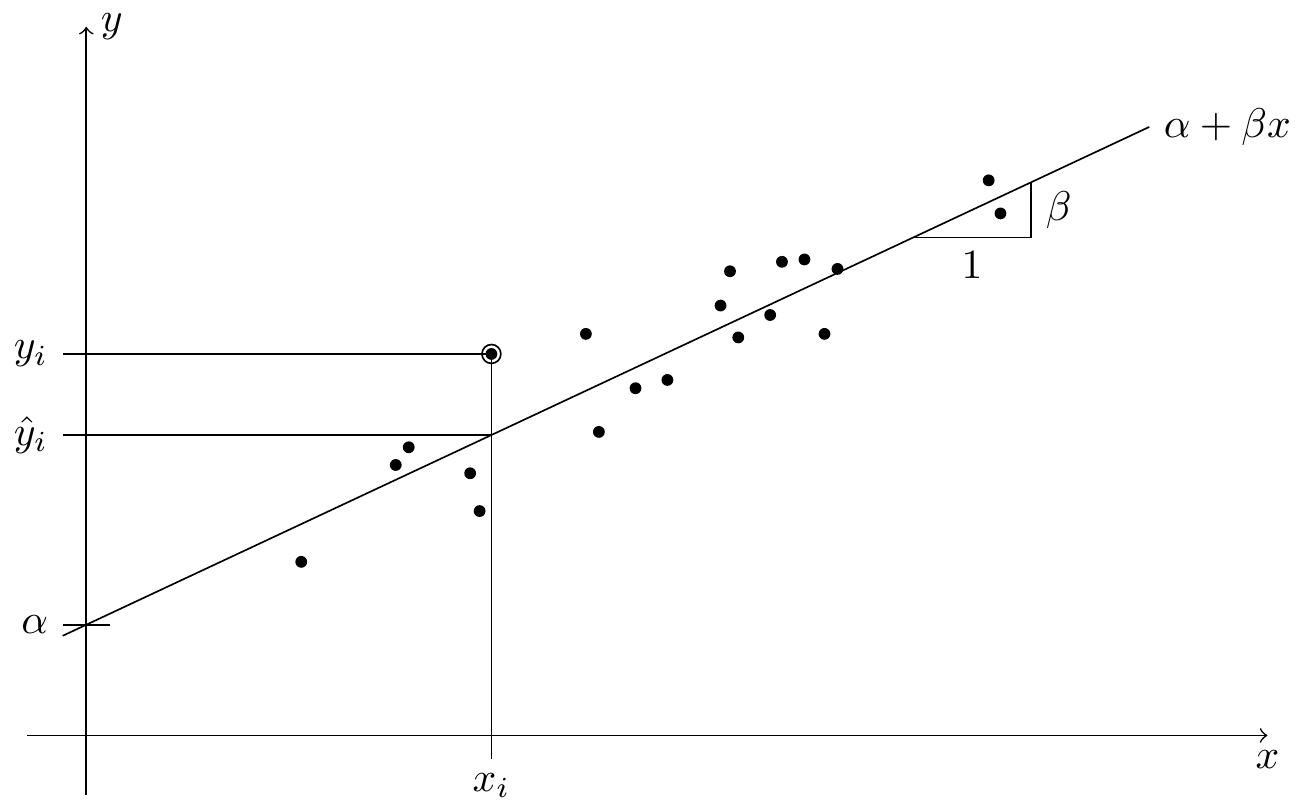# Preface

From previous modules we know how to fit a regression line through points $$(x_1, y_1), \ldots, (x_n, y_n) \in\mathbb{R}^2$$. The underlying model here is described by the equation $\begin{equation*} y_i = \alpha + \beta x_i + \varepsilon_i \end{equation*}$ for all $$i \in \{1, 2, \ldots, n\}$$, and the aim is to find values for the intercept $$\alpha$$ and the slope $$\beta$$ such that the residuals $$\varepsilon_i$$ are as small as possible. This procedure, called simple linear regression, is illustrated in figure 0.1.Figure 0.1: An illustration of linear regression. Each of the black circles in the plot stands for one paired sample $$(x_i, y_i)$$. The regression line $$x \mapsto \alpha + \beta x$$, with intercept $$\alpha$$ and slope $$\beta$$, aims to predict the value of $$y$$ using the observed value $$x$$. For the marked sample $$(x_i, y_i)$$, the predicted $$y$$-value is $$\hat y$$.

In this situation, the variable $$x$$ is called a input, feature, or sometimes the explanatory variable or the “independent variable”. The variable $$y$$ is called response or output, or sometimes the “dependent variable”, and $$\varepsilon$$ is called the residual or error.

Extending the situation of simple linear regression, in this module we will consider multiple linear regression, where the response $$y$$ is allowed to depend on several input variables. The corresponding model is now $\begin{equation*} y_i = \alpha + \beta_1 x_{i1} + \cdots + \beta_p x_{ip} + \varepsilon_i \end{equation*}$ for all $$i \in \{1, 2, \ldots, p\}$$, where $$n$$ is still the number of observations, and $$p$$ is now the number of inputs we observe for each sample.

Note that for multiple linear regression, we still consider a single response for each sample, only the number of inputs has been increased. One way to deal with situations where there is more than one output would be to fit separate models for each output.

We will discuss multiple linear regression in much detail; our discussion will be guided by three different aims of linear regression:

1. Prediction: given a not previously observed value $$x$$, try to predict the corresponding $$y$$.
2. In cases were the residuals $$\varepsilon_i$$ correspond to unwanted noise, the fitted values $$\hat y_i = \alpha + \beta x_i$$ can be considered to be de-noised versions of the observed values $$y_i$$.
3. By studying a fitted regression model, sometimes better understanding of the data can be achieved. For example, one could ask whether all of the $$p$$ input variables carry information about the response $$y$$.

We will address these aims by considering different questions, like how to estimate the coefficients $$\alpha, \beta_1, \ldots, \beta_p$$, how to assess model fit, or how to deal with outliers in the data.